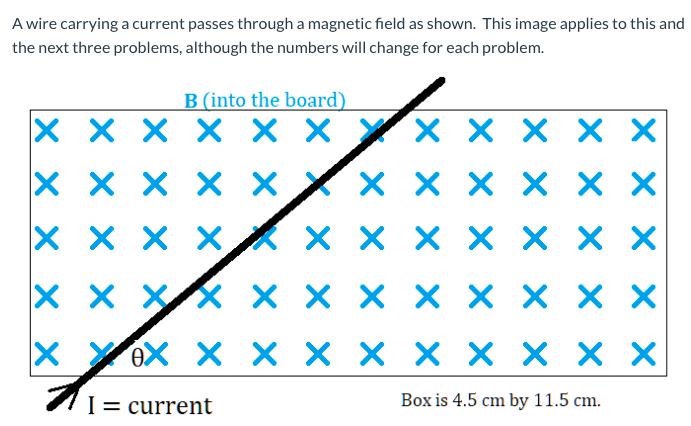# B (into the board) X x x x × × Yx × × × X x x x xk x x × x × × x x x XX X x X X X × × X eX X X X × × X X × X I= current Box is 4.5 cm by 11.5 cm.

Question

What is the length of the wire inside the magnetic field? Answer in centimeters.  (B = 0.001 T, θ = 32°, I = 5.71 A)help_outlineImage TranscriptioncloseB (into the board) X x x x × × Yx × × × X x x x xk x x × x × × x x x XX X x X X X × × X eX X X X × × X X × X I= current Box is 4.5 cm by 11.5 cm. fullscreen

### Want to see this answer and more?

Experts are waiting 24/7 to provide step-by-step solutions in as fast as 30 minutes!*

*Response times may vary by subject and question complexity. Median response time is 34 minutes for paid subscribers and may be longer for promotional offers.
Tagged in
Science
Physics

### Electromagnetic Induction